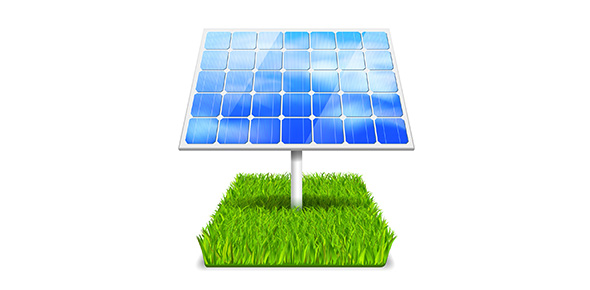# Centrifugal Pump Fundamentals #1

15 Questions | Attempts: 540
ShareSettingsThe following quiz tests on the general knowledge of the fundamental centrifugal pump concepts on pressure and basic abbreviations and what they stand for. Take the test to learn more on the centrifugal pump. All the best.

• 1.
Given a pressure gauge reading of 100 PSIG for a fluid that weighs 2 times as much as water yet with the same viscosity as water, calculate the Head of Water Equivalent.
• A.

150 ft

• B.

73.5 ft

• C.

115.5 ft

• D.

125 ft

• 2.
Water has filled a 2” vertical pipe that is 115.5 feet tall. If a gauge was installed at the bottom of the pipe, what would the gauge pressure be?
• A.

40 psi

• B.

50 psi

• C.

55 psi

• D.

60 psi

• 3.
In Q2, what is this “head” called?
• A.

• B.

• C.

• D.

TDH

• 4.
Given H = 100 ft, Flow = 200 GPM, Specific Gravity is 1.2, and efficiency is 60.6%, what is the Brake Horsepower?
• A.

10

• B.

12

• C.

15

• D.

17.5

• 5.
What does “BEP” stand for?
• A.

Basic Energy Parameter

• B.

Best Energy Potential

• C.

Best Efficiency Point

• D.

Black Eyed Peas

• 6.
Fill in the blank: A pump operates where it intersects the _________curve.
• A.

System

• B.

Pump

• C.

Tangential

• D.

Velocity

• 7.
For questions 7-14, use the pump curve picture from the email. Match the terms with the corresponding letters found on the curve: Impeller Trim
• A.

A

• B.

B

• C.

D

• D.

F

• 8.
GPM (flow rate)
• A.

C

• B.

D

• C.

E

• D.

G

• 9.
TDH
• A.

A

• B.

C

• C.

E

• D.

G

• 10.
Efficiency Lines
• A.

A

• B.

B

• C.

C

• D.

D

• 11.
Horsepower
• A.

A

• B.

B

• C.

C

• D.

D

• 12.
NPSHr
• A.

A

• B.

G

• C.

F

• D.

C

• 13.
BEP
• A.

A

• B.

C

• C.

F

• D.

G

• 14.
What would be nearest answer for the “shut-off head” for this pump with a 9” impeller trim?
• A.

105 ft

• B.

90 ft

• C.

80 ft

• D.

70 ft

• E.

50 ft

• 15.
Fill in the blanks - (a) and (b) : TDH = __(a)__ Head - ___(b)___ Head
• A.

A) Top; b) Bottom

• B.

A) Bottom; b) Top

• C.

A) Suction; b) Discharge

• D.

A) Discharge; b) SuctionBack to top
×

Wait!
Here's an interesting quiz for you.